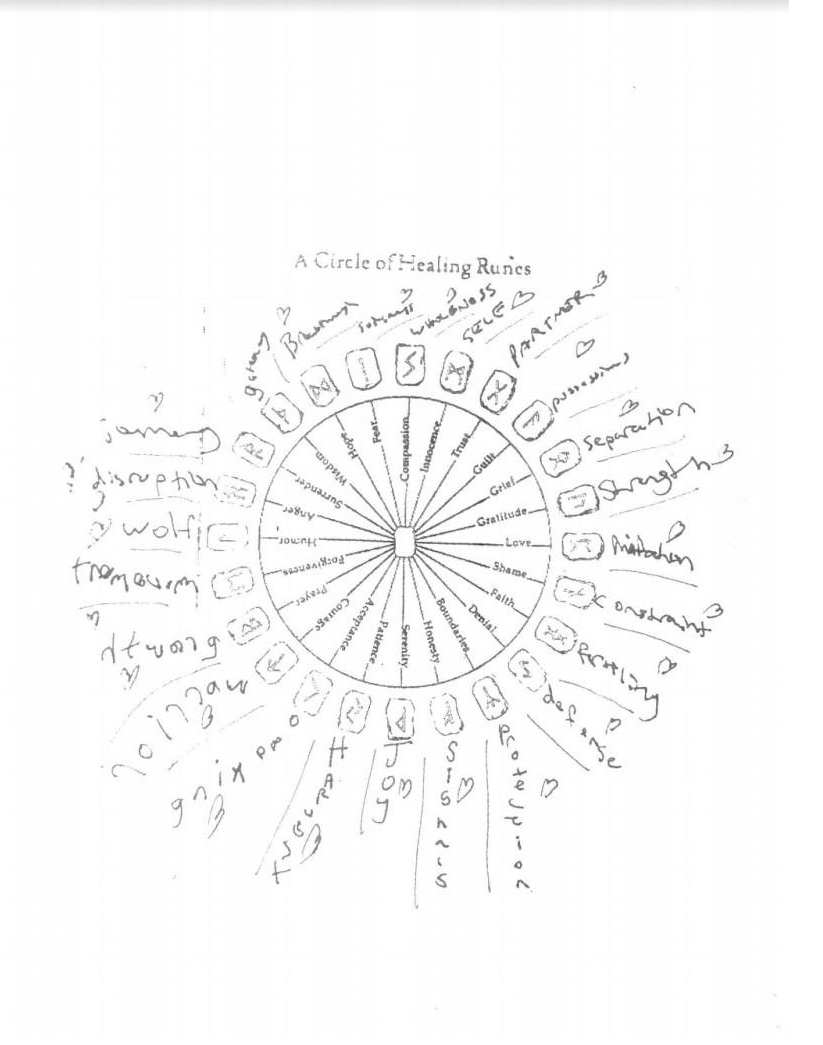))))))))))))))))))))))))))):::Star War White 1:3:6 ::::
```White white white

White
Red
Orange
Yellow
Blue
Indigo
Violet
Black
HomeOh when is the when when when?
Win in the win win wins!
Zero sum is not nero sum
Her sum is near sums for sums for all sums
All sums is one sum
One sum is divided amongst many smaller sums
These are amas, in the All
Are there Alls but the All?
Are there amas, not, yet, but upon more amas, and amas within amas, amas about amas, amas around amas, amas always stalking amas, amas, egos, ego projections.
))))))))))))))))))))))))))):::Star War White 1:3:6 ::::

```